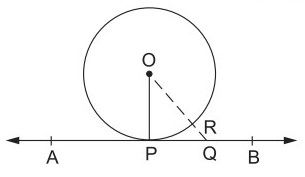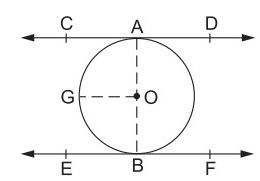top of pageSearch

# CIRCLE THEOREM

Updated: May 8, 2020

Theorem 1.The tangent at any point of a circle is perpendicular to the radius through the point of contact.GIVEN A circle with centre O and a tangent AB

at a point P of the circle.

TO PROVE:- OP AB

CONSTRUCTION Take a point Q,other than P,on AB. Join OQ.

PROOF Q is a point on the tangent AB, other than the point of contact P.

∴ Q lies outside the circle.

Let OQ intersect the circle at R.

Then, OR < OQ [a part is less than the whole] .… (i)

But, OP= OR [radii of the same circle]. .… (ii)

OP < OQ [from (i) and (ii)].

Thus, OP is shorter than any other line segment joining O to any

point of AB, other than P.

In other words, OP is the shortest distance between the point O

and the line AB.

But, the shortest distance between a point and a line is the

perpendicular distance.

OPAB .

REMARKS (i) From this theorem we also conclude that at any point on a

circle, one and only one tangent can be drawn to the circle.

(ii) The line containing the radius through the point of contact

is called the ’normal‘ to the circle at the point of contact.

Theorem 2.(Converse of Theorem 1) A line drawn through the end of a radius

and perpendicular to it is a tangent to the circle.GIVEN A circle with centre O in which OP is

a radius and AB is a line through P

such that OP ⊥ AB .

TO PROVE AB is a tangent to the circle at the point P.

CONSTRUCTION Take a point Q, different from P, on AB. Join OQ.

PROOF We know that the perpendicular distance from a point to a line is

the shortest distance between them.

∴ OP ⊥ AB ⇒ OP is the shortest distance from O to AB.

∴ OP < OQ .

∴ Q lies outside the circle [∵ OP is the radius and OP < OQ].

Thus, every point on AB, other than P, lies outside the circle.

∴ AB meets the circle at the point P only.

Hence, AB is the tangent to the circle at the point P.

REMARK This theorem gives us a method of constructing a tangent at a

point P on the circle. We first draw the radius OP and then draw PT ⊥ OP . Then, PT is the tangent at P

Theorem 3.The lengths of tangents drawn from an external point to a circle

are equal.GIVEN Two tangents AP and AQ are drawn from a point A to

a circle with centre O.

TO PROVE AP = AQ.

CONSTRUCTION Join OP, OQ and OA.

PROOF AP is a tangent at P and OP is the radius through P.

OP AP .

Similarly, OQ AQ .

In the right OPA and OQA, we have:

OP = OQ [radii of the same circle]

OA = OA [common]

ΔOPA ≅ ΔOQA , [by RHS-congruence].

Hence, AP = AQ [cpct].

REMARK The above theorem can also be proved by using the Pythagoras’

theorem:

AP = OA² - OP² = OA² - OQ² = AQ [ OP = OQ = radius]

AP = AQ .

Theorem 4.If two tangents are drawn from an external point then

(i) they subtend equal angles at the centre, and

(ii) they are equally inclined to the line segment joining the centre

to that pointGIVEN A circle with centre O and a point A outside it. Also, AP and AQ are the two

tangents to the circle.

TO PROVE ∠AOP = ∠AOQ and ∠OAP = ∠OAQ .

PROOF In ΔAOP and ΔAOQ, we have

AP = AQ [tangents from an external point are equal]

OP = OQ [radii of the same circle]

OA = OA [common]

ΔAOP ΔAOQ , [by SSS-congruence].

Hence, ∠AOP = ∠AOQ and ∠OAP = ∠OAQ .

REMARK Since ∠OAP = ∠OAQ , i.e., AO is the bisector of ∠PAQ, so the centre

lies on the bisector of the angle between the two tangents.

Theorem 5.Prove that in two concentric circles, the chord of the larger circle,

which touches the smaller circle, is bisected at the point of contact.GIVEN Two circles with the same centre O and AB is a

chord of the larger circle touching the smallercircle at C.

TO PROVE AC = BC .

CONSTRUCTION Join OC.

PROOF AB is a tangent to the smaller circle at the point C and OC is the

OC AB .

But, the perpendicular drawn from the centre of a circle to a chord

bisects the chord.

OC bisects AB [ AB is a chord of larger circle].

Hence, AC = BC .

Theorem 6.Prove that the tangents drawn at the ends of a diameter of a circle are parallel.GIVEN CD and EF are the tangents at the end points

A and B of the diameter AB of a circle withcentre O.

TO PROVE CD EF .

PROOF CD is the tangent to the circle at the point A

EF is the tangent to the circle at the point B.

EF OB ⇒ ∠OBE = 90ᴼ ⇒ ∠ABE = 90ᴼ .

Thus,BAD = ABE (each equal to 90).

But these are alternate interior angles.

CD EF .

Theorem 7.Prove that the line segment joining the points of contact of two

parallel tangents to a circle is a diameter of the circle.GIVEN CD and EF are two parallel tangents at the points A and B of acircle with centre O.TO PROVE AOB is a diameter of the circle.

CONSTRUCTION Join OA and OB. Draw OG CD.

PROOF OG CD and AO cuts them.

CAO + GOA = 180

90 + GOA = 180ᴼ [ OA CD]

GOA = 90.

Similarly,∠GOB =90ᴼ.

GOA + GOB = 90 + 90 = 180

AOB is a straight line.Hence, AOB is a diameter of the circle with centre O.

Theorem 8.Prove that the angle between the two tangents drawn from an external point to a circle is supplementary to the angle subtended by

the line segments joining the points of contact to the centreGIVEN PA and PB are the tangents drawn from a point P to a circle with

centre O. Also, the line segments OA and OB are drawn.

TO PROVE APB + AOB = 180 .

PROOF We know that the tangent to a circle is

the point of contact.

PA OA OAP = 90 , and

PB OB OBP = 90 .

OAP + OBP = 90 + 90 = 180 . … (i)

But, we know that the sum of all the angles of a quadrilateral is 360

∴ ∠OAP + OBP + APB + AOB = 360 . … (ii)

From (i) and (ii), we get

APB + AOB = 180

Theorem 9.Prove that there is one and only one tangent at any point on the

circumference of a circle.Let P be a point on the circumference of a circle with centre O.

If possible, let PT and PT' be two tangents at a point P of the circle.

Now, the tangent at any point of a circle is perpendicular to the

radius through the point of contact.

OP PT and similarly, OP PT'

⇒ ∠OPT = 90 and OPT' = 90.

⇒ ∠OPT = OPT'

This is possible only when PT and PT' coincide.

Hence, there is one and only one tangent at any point on the

circumference of a circle.

∠ ⊥ Δ ⇒ ∴ ∵ ∥ ᴼ

Theorem 10.Prove that the perpendicular at the point of contact of the tangent to

a circle passes through the centre.Let PT be a tangent to a circle with centre O,

where P is the point of contact.

Let PQ PT = , where Q lies on the circle, i.e.,

QPT = 90 .

If possible, let PQ not pass through the

centre O.

Join PO and produce it to meet the circle at R.

Then PO being the radius through the point of contact, we have

PO PT = [ the tangent is perpendicular to the radius

through the point of contact]

OPT = 90 ⇒∠RPT = 90 .

Thus, we have QPT = RPT = 90 .

This is possible only if P, Q and R are collinear.

But a straight line cuts a circle in at the most two points.

So, the points Q and R coincide.

Hence, PQ passes through the centre O, i.e., the perpendicular at

the point of contact to the tangent passes through the centre.